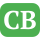CppBuzz.com

# Type Casting in C++ (static, dynamic, reinterpret & const_cast)

Four type of casting operators in C++ are listed below

• static_cast [convert non polymorphic types]
• const_cast [add or remove the const-ness or volatile-ness type]
• dynamic_cast [convert polymorphic types]
• reinterpret_cast [type conversion of unrelated types]

## Example of static_cast

```#include <iostream.h>
#include <stdlib.h>

int main()
{
int sum = 1000;
int count = 21;
double average1 = sum/count;
cout<<"Before conversion = "<<average1<<endl;
double average2 = static_cast<double>(sum)/count;
cout<<"After conversion = "<<average2<<endl;
system("pause");
return 0;
}
```

## Example of const_cast

```//demonstrates const_cast
#include <iostream.h>
#include <stdlib.h>

int main()
{
/* p = 10 is a constant value,
cannot be modified*/

const int p = 20;
cout<<"const p = ";
cout<<p;
cout<<"\nq = p + 20 = ";
cout<<(p + 20)<<endl;

/*The following code should generate error,
because we try to modify the constant
value... uncomment, recompile and re run,
notice the error...
p = 15;
p++;
remove the const...
*/

int r = const_cast<int&> (p);

/*the value of 10 should be
modified now...*/

--r;

//Removing the const, decrement by 1
cout<<"\nNew value = "<<r<<endl;
system("pause");
}
```

## Example of Dynamic cast

```//upcast conversion using dynamic_cast

#include <iostream.h>
#include <stdlib.h>

//base class
class Base1 {};

//derived class...
class Derived1:public Base1 {};

//another derived class
class Derived2:public Derived1{};

//dynamic_cast test function...
void funct1()
{
//instantiate an object…
Derived2* Test1 = new Derived2;

/*
upcasting, from derived class to base class,
Derived1 is a direct from Base1
making Test2 pointing to Derived1 sub-object of Test1
*/

Derived1* Test2 = dynamic_cast<Derived1*>(Test1);
cout<<"Derived1* Test2 =
dynamic_cast<Derived1*>(Test1);"<<endl;

if(!Test2)
cout<<"The conversion is fail..."<<endl;
else
cout<<"The conversion is successful..."<<endl;

//upcasting, from derived class to base class
//Derived2 is an indirect from Base1
Base1* Test3 = dynamic_cast<Derived1*>(Test1);
cout<<"\nBase1* Test3 =
dynamic_cast<Derived1*>(Test1);"<<endl;

if(!Test3)
cout<<"The conversion is fail..."<<endl;
else
cout<<"The conversion is successful..."<<endl;
}

int main()
{
funct1();
system("pause");
return 0;
}
```

## Example of reinterpret_cast

```//unsigned int pointers conversion

#include <iostream.h>
#include <stdlib.h>
unsigned int* Test(int *q)
{
//convert int pointer to unsigned int pointer
unsigned int* code=reinterpret_cast<unsigned int*>(q);

/*
return the converted type data,
a pointer...
*/
return code;
}

int main(void)
{
//array name is a pointer...
int a;
cout<<"int pointer unsigned int pointer";

for(int i = 0;i<=10;i++)
cout<<(a+i)<<" converted to:";

cout<<Test(a+i);
system("pause");
return 0;
}

```